### Insert math elements

An equation contains expressions made up of math elements—characters, text strings, operators, and other mathematical elements from the Equations pod.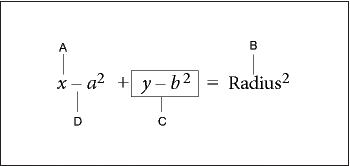A. Alphanumeric character B. Text string C. Expression D. Operator

After you create an equation object, insert math elements in it by typing them or by clicking them on the Equations pod. The Equations pod can be closed when you insert an item from the keyboard.

note:   Math equations support the Unicode text-encoding standard.

#### About the scope of operations

When you insert a math element in an equation, FrameMaker adjusts the mathematical syntax. The result depends on the scope of operation—the selection or the location of the insertion point. For example, when you insert x in a fraction, the result depends on whether the numerator, the denom­inator, or the entire fraction is selected.

If you multiply by x

The result is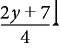or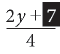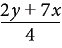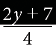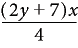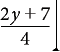or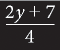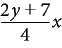In the first example, only the 7 is multiplied by x. In the second example, FrameMaker adds paren­theses to show that the entire expression 2y + 7 is multiplied by x. In the last example, the entire frac­tion is multiplied by x. The size of the insertion point in the first and last examples indicates the scope.

Many mistakes in equations result from an incorrect scope of operation—having too little or too much selected when inserting an element. Be careful to select the range you want before inserting an element.

#### Insert a math element

1)Select an expression or place the insertion point in an equation.

2)Do any of the following:

To insert a math element by using the Equations pod, click the element.

To insert an alphanumeric character or a symbol that appears on the keyboard, type it. You can type parentheses, brackets, plus signs, equal signs, and so on. If you type an open parenthesis, FrameMaker inserts the closing parenthesis.

To insert an element by typing a backslash sequence, type a backslash ( \ ) and the string that identifies the element, and then press Return. For example, to insert the symbol for infinity, type\infty  and press Return.

Insert a custom math element.

If an element needs one or more operands, a question mark prompt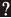appears for each operand; otherwise, an insertion point appears. To move from one operand to another, press Tab.

#### Insert a text string

A text string is a series of characters that is interpreted as a unit. Unlike characters that make up an expression, the characters in a text string don’t usually appear in italics and aren’t multiplied together.

A. Text strings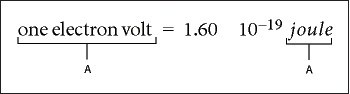Text strings have no mathematical meaning in FrameMaker. For example, evaluation commands can evaluate the function tan but not the string “tan”.

1)On the Symbols page of the Equations pod, click Start String. Two double quotation marks ( " " ) appear. You can also type an apostrophe ( ' ) or a double quotation mark (") to start the string.

2)Type the text of the string. The quotation marks disappear, and the text appears as you type. To insert a straight apostrophe or straight double quotation mark as part of the string, hold down Control while pressing the key.

3)Click End String on the Symbols page or press Return.

#### Insert, add, or edit a horizontal or vertical list of expressions

An equation object usually contains one equation or expression. You can set up a horizontal or vertical list in which several expressions are contained in the same object. When you select a list, all the expressions in the list are selected as one object.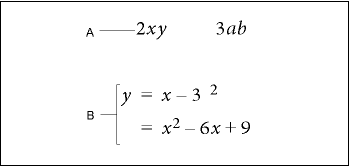A. Horizontal list B. Vertical list aligned on equal signs

FrameMaker aligns the expressions in the list automatically. When you move the list, all the expres­sions maintain their relative positions.

1)Select the expression you want to be the first item in the list or to add to a list.

2)On the Operators page of the Equations pod, click the horizontal list element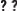or vertical list element. To add a math element, type the elements or click items on the Equations pod. A horizontal or vertical list appears. The selected expression appears as the first item in the list. A second item appears in the list as a question mark prompt.

Before clicking

After clicking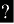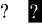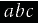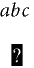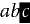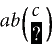In the first example, a horizontal list is inserted. In the second example, a vertical list is inserted, and the selected expression becomes the first item in the list. In the last example, a vertical list is inserted as part of a larger expression.

#### Insert symbols

The Symbols page on the Equations pod contains the Greek alphabet, other symbols, and diacritical marks.

The Symbols page doesn’t include Greek letters that have Roman-alphabet equivalents, such as the uppercase alpha (A). The following examples show the result of clicking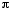on the Symbols page.

Before clicking

After clicking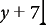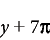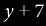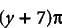Diacritical marks appear on the element to the left of the insertion point or on the selected expres­sion. Click the dot or prime button once for each dot or prime diacritical mark you want to insert.

note:   To interpret and mathematically manipulate a summation, product, or partial differential element, insert the element from the Large page or Calculus page. Do not insert from the Symbols page. Elements from the Symbols page are not evaluated.

#### Insert operators

The Operators page on the Equations pod contains commonly used operators, roots, powers, signs, subscripts, superscripts, and logic symbols.

If an expression is selected when you insert the operator, the expression becomes the first operand. The following examples show the result of clicking the plus sign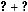or the division operatoron the Operators page.

Before clicking

After clicking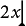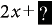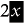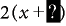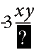You can represent the division operator as a slash (/), a horizontal bar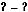, or a division sign.

FrameMaker can evaluate exponents mathematically, but not superscripts.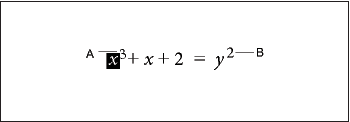A. Superscript B. Exponent

#### Insert large elements

The Large page on the Equations pod contains sums, products, integrals, intersections, and unions.

If an expression is selected when you insert a large element, the expression becomes the first operand. The following examples show the result of clicking the summation symbol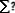on the Large page.

Before clicking

After clicking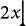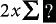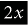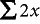You can add an operand after inserting a math element from the Large page.

#### Insert delimiters

The Delimiters page contains delimiters such as parentheses, brackets, and curly brackets.

If an expression is selected when you insert a pair of delimiters, the expression is placed inside the delimiters. The following examples show the result of clicking the parentheses on the Delimiters page.

Before clicking

After clicking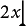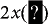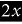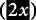You can also represent parentheses as brackets ([ ]) or curly brackets ({ }).

#### Insert relations

The Relations page contains relation symbols such as =, <, >,,,,, and.

All relation symbols—except the binary equal sign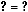—apply to the character to the left or right of the insertion point or to the selection. When you insert a relation symbol between multiplied elements, it applies to the elements on either side of the insertion point.

Binary equal signsappear to the right of the current expression (as shown in the second example). When the insertion point or selection is in a subscript or superscript, the equal sign appears in the subscript or superscript.

Before clicking

After clicking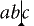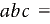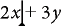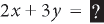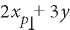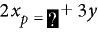##### Insert a binary equal sign at the insertion point

1)Press Esc m =

Before clicking

After clicking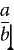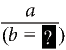#### Insert calculus elements

The Calculus page contains integrals, derivatives, partial derivatives, gradients, and the limit func­tion.

If an expression is selected when you insert the calculus element, the expression becomes the first operand. The following examples show the result of clicking the integral symbolon the Calculus page.

Before clicking

After clicking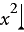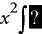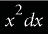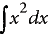You can change the position of operands and add operands.

#### Insert matrices

The Matrices page contains elements for matrices ranging in size from 1 by 1 to 3 by 3. After creating a matrix, you can add rows and columns.

If an expression is selected when you insert the matrix element, the expression becomes the first cell in the matrix.

Before clicking

After clicking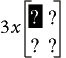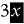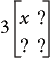#### Insert functions

The Functions page contains trigonometric, hyperbolic, and logarithmic functions, and also the sign, limit, and general functions.

FrameMaker places functions at the insertion point or at the selection. When an expression is selected, the expression becomes the argument of the function—except for the limit and general functions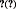and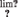. The following examples show the result of clicking the sin function on the Functions page.

Before clicking

After clicking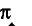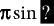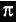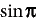#### Insert a custom math element

In addition to inserting math elements that are built in FrameMaker, you can insert custom math elements that have been defined for a document.

Custom math elements have no mathematical meaning in FrameMaker. For example, a custom math element for an operator is treated as an operator in an equation. However, FrameMaker cannot evaluate it.

1)On the Equations pod, choose Insert Math Element from the Equations pop-up menu.

2)Select the element name and click Insert.

September 30, 2016